• No products in the cart.

# 203.3.6 The Decision Tree Algorithm

### The Decision tree Algorithm

In previous section, we studied about Information Gain in Decision Tree Split

• The major step is to identify the best split variables and best split criteria
• Once we have the split then we have to go to segment level and drill down further

Until stopped:

1. Select a leaf node
2. Find the best splitting attribute
3. Spilt the node using the attribute
4. Go to each child node and repeat step 2 & 3

Stopping criteria:

• Each leaf-node contains examples of one type
• Algorithm ran out of attributes
• No further significant information gain

### The Decision tree Algorithm – Demo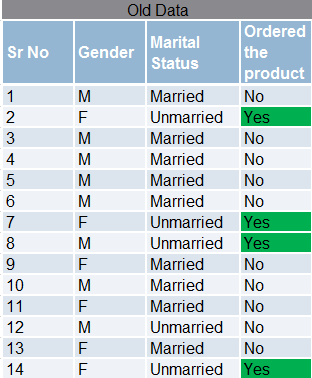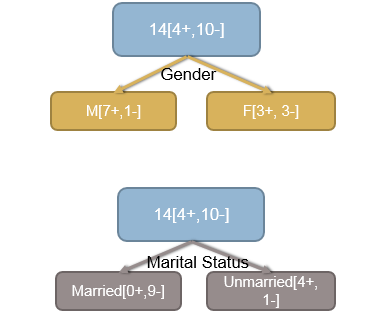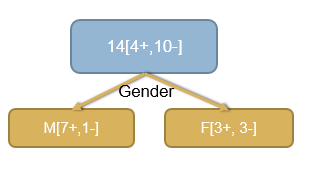Entropy([4+,10-]) Ovearll = 86.3% (Impurity)

• Entropy([7+,1-]) Male= 54.3%
• Entropy([3+,3-]) Female = 100%
• Information Gain for Gender=86.3-((8/14)54.3+(6/14)100) =12.4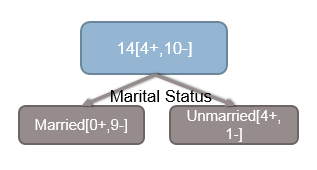Entropy([4+,10-]) Ovearll = 86.3% (Impurity)

• Entropy([0+,9-]) Married = 0%
• Entropy([4+,1-]) Un Married= 72.1%
• Information Gain for Marital Status=86.3-((9/14)0+(5/14)72.1)=60.5
• The information gain for Marital Status is high, so it has to be the first variable for segmentation• Now we consider the segment “Married” and repeat the same process of looking for the best splitting variable for this sub segment ### The Decision tree Algorithm

Until stopped: 1. Select a leaf node 2. Find the best splitting attribute 3. Spilt the node using the attribute 4. Go to each child node and repeat step 2 & 3 Stopping criteria: – Each leaf-node contains examples of one type – Algorithm ran out of attributes – No further significant information gain

### Many Splits for a Single Variable

• Sometimes we may find multiple values taken by a variable
• which will lead to multiple split options for a single variable
• that will give us multiple information gain values for a single variable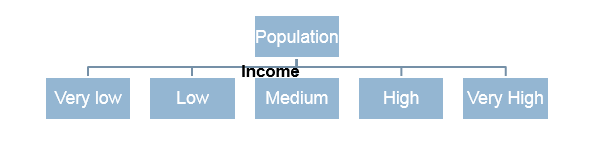What is the information gain for income?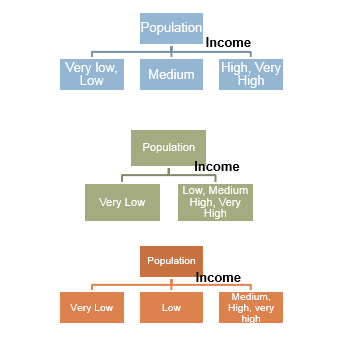• What is the information gain for income?
• There are multiple options to calculate Information gain
• For income, we will consider all possible scenarios and calculate the information gain for each scenario
• The best split is the one with highest information gain
• Within income, out of all the options, the split with best information gain is considered
• So, node partitioning for multi class attributes need to be included in the decision tree algorithm
• We need find best splitting attribute along with best split rule

### The Decision tree Algorithm- Full version

Until stopped: 1. Select a leaf node 2. Select an attribute – Partition the node population and calculate information gain. – Find the split with maximum information gain for this attribute 3. Repeat this for all attributes – Find the best splitting attribute along with best split rule 4. Spilt the node using the attribute 5. Go to each child node and repeat step 2 to 4

Stopping criteria:

• Each leaf-node contains examples of one type
• Algorithm ran out of attributes
• No further significant information gain

The next post is about Building a Decision Tree in R.

20th June 2017

### 0 responses on "203.3.6 The Decision Tree Algorithm"

Statinfer Software Solutions LLP

Software Technology Parks of India,
NH16, Krishna Nagar, Benz Circle,